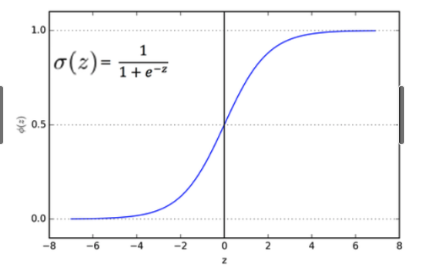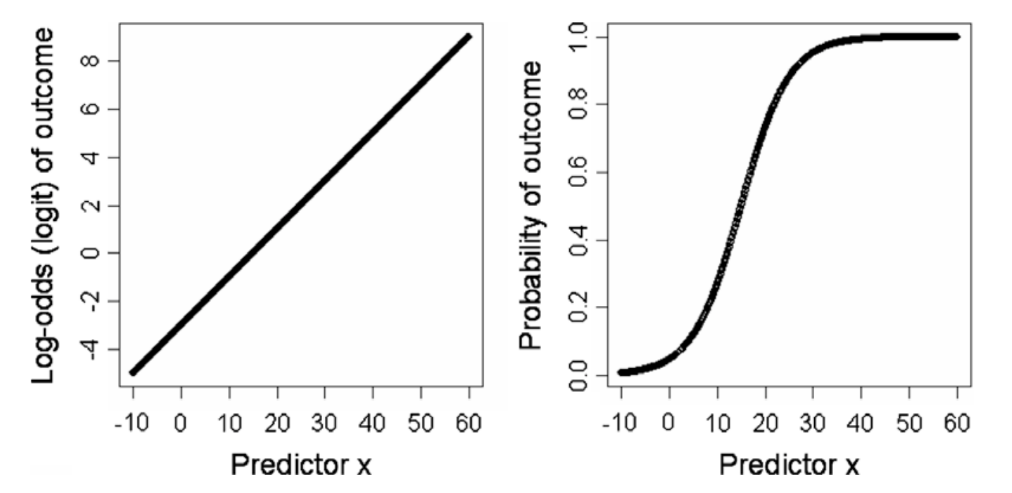# Logistic Regression

kobeisfree94·2022년 6월 16일
0

## Classification

Objective:

• Study/observe the relationship between categories of the preexisting data in order to evaluate/predict new data into proper categories.
• Different from Regressions in that:
- Regression -- uses Mean
- Classification -- uses Accuracy = TP + TN / P+N
- Uses the majority class(mode) as the baseline

Example:

target = 'Survived'
y_train = train[target]
y_train.value_counts(normalize = True)
#0 0.625749
#1 0.374251
#Name: Survived, dtype: float 64
major = y_train.mode()
y_pred = [major] + len(y_train)

from sklearn.metrics import accuracy_score
print('training accuracy', accuracy_score(y_train, y_pred))
#training accuracy : 0.625738

## Logistic Regression

• Used when the Dependent Variable is a Nominal Variable
• Has a similar shape as the Linear Regression
• BUT is used when the dependent variable is binomial or a polynomial

Calculates the probability of whether the observed data belongs in a particular category/class.#### Logit Transformation

• Transforms data by applying log to the odds
• Odd = The ratio of Success(1) to Failure(0)
- odds = 4, probability of success if 4x that to failure
• Objective: Makes understanding the logistic regression easier
• Observe the changes in logits by looking at how much Predictor X increasesUses in Real Life:

• Credit Evaluation
• Cancer Diagnosis
• Fraud Detection
• Spam Mail Classification

Weaknesses of Logistic Regression

• Difficult to deal with ordinal regression
• Difficult to apply to non-linear problems
• Difficult to explain the exact relationship(?)